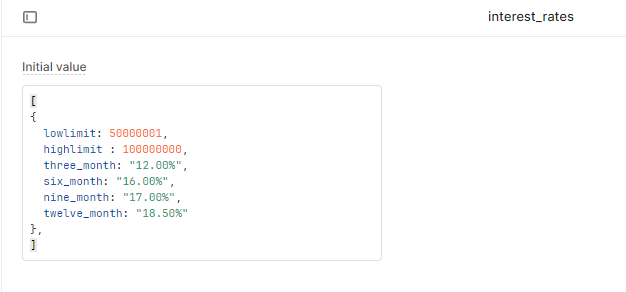# I need help to get this done

I am trying to make `interestRate` field generate a value based on the two input fields `investmentAmount` and `tenor`. There is a Google Sheet that has the data in a table. I just want to modify a form in a way that when a value is input in the `investmentAmount` field and a certain value is selected in the `tenor` field, it generates the rate based on the range the `investmentAmount` falls under and the tenor chosen, in accordance to the table.

Lastly, I want to be able to write a formula to calculate the "Total interest to be paid" based on the `investmentAmount`, `Tenor` and `interestRate`. I am a Newbie, please help.

With the small amount of data you have for the investment range in google sheet, it can be written in a temporary state variable in Retool without needing to connect to google sheet (it takes a bit of work setting it up if you want to make the connection).

I suggest to go with temporary state variable for the time being, it would make things a lot easier.Here I have created a temporary state variable as JSON data similar to what you would have in the google sheet.

I have created a few Inputs and created a query to update the interest rate % on tenor change, the event handler in the tenor component will trigger the calculateRates query.

testApp.json (10.3 KB)
I have attached the app that I created, you can import it and see how it works. Populate the interest_rate data and test it out. Afterwards you can try to calculate the total interest to be paid.

2 Likes

Hi @lenti
Thank you so much. It worked. One last thing is: I am trying to make the total_interest field to generate a value based on the multiplication of the `amount` and `rate` field. Please help.

Using the calculateRates query I created, you can replace it with this.

``````interest_rates.value.map((rate) => {
if (amount.value > rate.lowlimit && amount.value < rate.highlimit) {
let getTenor = rate[tenor.value];
rates.setValue(getTenor);
let mapMonth = {
three_month: 3,
six_month: 6,
nine_month: 9,
twelve_month: 12,
};
/*
A = P(1+RT)
A = total accrued amount
P = principal
R = interest rate
T = time period
*/
let P = amount.value;
let R = parseFloat(rates.value) / 100.0;
let T = (mapMonth[tenor.value] * 30) / 365;
let A = parseInt(P * (1 + R * T));

//replace total_interest with the textInput component where you wanted the result.
total_interest.setValue(A-P);
}
});

``````

The total interest formula I used maybe a bit off, feel free to change it.

3 Likes